﻿ Single-objective Optimization of Passive Shock Absorber for Landing GearPublications are Open
Access in this journal
Article Versions
Export Article
• Normal Style
• MLA Style
• APA Style
• Chicago Style
Research Article
Open Access Peer-reviewed

### Single-objective Optimization of Passive Shock Absorber for Landing Gear

Fenghui Shi , Warren Isaac Anak Dean, Taikei Suyama
American Journal of Mechanical Engineering. 2019, 7(3), 107-115. DOI: 10.12691/ajme-7-3-1
Received April 23, 2019; Revised June 08, 2019; Accepted June 17, 2019

### Abstract

In previous paper, “Multi-objective optimization of Passive Shock Absorber for Landing Gear”, several new construction of metering pin in passive shock absorber have been proposed, and obtained optimum solutions of metering pin dimensions by multi-objective optimization. The aim of the previous paper is to solve the mass variation problem. The shock absorbers with the results of multi-objective optimization for the new metering pin constructions can be called a “semi semi-active shock absorber”. In this paper, we do single-objective optimization just for simple passive shock absorbers, namely optimization at maximum or minimum masses. The aim of this paper is to evaluate the performance of simple passive shock absorbers with new construction metering pin, not as a semi semi-active shock absorber to deal with the mass variation problem. We proposed single-objective optimization method of passive shock absorber without metering pin, with single and multi-tapered metering pins, and with single and multi-parabolic metering pins. Each of these metering pin constructions have been obtained optimal solutions. Using the optimum solutions, we evaluate and compare the performance of each passive shock absorber without and with different metering pins for maximum or minimum masses. The optimization results and method are helpful in the design of landing gears for various aircrafts, especially, for design of lighter aircraft landing gears, not necessary to be considered mass vibration problem.

### 1. Introduction

Landing gear shock absorbers, usually oleo pneumatic shock absorbers, are intended for the reduction of forces transmitted to the aircraft from the impact during landing, as well as from the disturbance due to the unevenness of the runway. Passive shock absorbers are commonly used in aircraft landing gears, and can be optimally designed for specific conditions. However, passive shock absorbers cannot function optimally under conditions that differ from their specified conditions. We call this the mass variation problem of passive shock absorbers. In order to solve these problems, since the 1970s, the active control and semi-active control began to be studied and tried to be used in the vibration control of the constructions of vehicle suspensions. Active and semi-active landing gears can provide good performance for both landing impact and taxi situations, while having the ability to adapt to various ground and operational conditions.

In Reference 1, a landing gear system featuring electrorheological/magnetorheological fluids was theoretically evaluated. In References 2 and 3, the active landing gear was experimentally compared to a passive landing gear. In References 4, the semi-active landing gear was designed using multi-objective optimization which has been applied in various studies 5. In Reference 6, the possible optimization strategies for semi-active landing gear at touchdown and during taxiing were discussed. In addition, problems concerning mass variation have been discussed 7, 8, 9, 10, 11. In References 11, a bypass shock absorber was discussed and tried to deal with the mass variation problem.

However, active and semi-active shock absorbers have complex constructions and require a complex control system. They also have high costs, and breaking risks. It is especially difficult to install active or semi-active shock absorbers in smaller aircrafts.

On the other hand, oleo pneumatic shock absorbers are highly efficient as they can absorb and remove vertical kinetic energy simultaneously. The main resistance during landing is the dynamic resisting force depending on the orifice area. Although the orifice is merely a hole in the orifice plate, most designs have a metering pin extending through it, and by varying the pin diameter, the orifice area will also vary. This variation is adjusted so that the strut load is constant under dynamic loading.

In order to solve the mass variation problem of aircraft landing gears (large and middle aircraft), we proposed several types constructions of metering pin in previous paper, “Multi-objective Optimization of Passive Shock Absorber for Landing Gear” 12. By changing the diameters of metering pins to reduce the mass variation problem, to make the same performance as the active and semi-active shock absorbers to a certain extent. Maybe they are can be called “semi semi-active shock absorbers”.

In this paper, we do single-objective optimization just for a simple passive shock absorber, namely optimization at maximum or minimum masses. The aim of this paper is to evaluate the performance of simple passive shock absorbers with new construction metering pin proposed in previous paper 12, not as a semi semi-active shock absorber to deal with the mass variation problem, and to compare the performance of conventional passive shock absorber and with new construction metering pin shock absorbers.

### 2. Passive Shock Absorber

In this study, we deal with shock absorbers without (Figure 1) and with metering pins (Figure 2) as same as the previous paper 12. The shock absorbers that consist of steel cylinder 1 and rod 2 which move in the cylinder in two guide bearings 3 and 4. Guide bearing 3 is rigidly attached to the rod and moves together with it, and guide bearing 4 is attached to the cylinder. Between the inner surface of the cylinder 1 and the outer surface of the rod 2, there is sealing ring 5.

The damping fluid partially fills the system as shown in Figure 1 and Figure 2. Above the liquid, at the top part of the cylinder, there is a gas, which is compressed by the liquid when the rod moves into the cylinder and elastically resists this motion. Hence, this shock absorber combines a hydraulic damper and a pneumatic spring into one unit. The column of the fluid moving in the cylinder together with the rod plays the role of the piston of the pneumatic spring.

When the external force is applied to the shock absorber, for example, by the wheels of the landing gear, the rod moves into the cylinder, and the damping fluid partially fills the chamber, compressing the gas. This leads to the elastic reaction of the pneumatic spring. As the rod moves, the fluid flows through the holes in the tube plunger producing the damping effect.

A metering pin with a variable cross-section is attached to the rod (Figure 2). As the rod moves, the metering pin moves through the hole and changes the clearance through which the fluid can flow. By appropriately profiling the metering pin diameters along its length, we can provide the required relationship for orifice areas. Technically, the variability of the area of the holes through which the damping fluid flows is adjusted so that the area of the holes and the resisting forces will be controlled depending on the change in mass of the aircraft.

• Figure 1. Schematic diagrams of passive shock absorber without metering pin
• Figure 2. Schematic diagrams of passive shock absorber with metering pin
• Figure 3. Schematic diagrams of the metering pin constructions

The guide bearing attached to the rod has holes through which the damping fluid can flow into the space between the cylinder and the rod. Inside the cylinder, there is tube plunger 6 with holes for the damping fluid to flow through. The plunger is attached to the top lid of the cylinder. Between the internal surface of the cylinder and rod, back valves 7 are placed to hamper the flow of the liquid into this space during the backward movement of the rod, thus increasing the damping effect

In this study, we considered metering pins constructions (Figure 3) as same as the previous paper 12 for optimization to find their optimal diameters, and compare shock absorbers without and with different metering pins.

### 3. Aircraft Landing Gear Model

3.1. Aircraft Weights

We deal with the Japanese national line Boeing 747-400 as the model. According to the JAL Practical Dictionary Flight Plan, Aircraft Specifications and Ability Table 13, the maximum design landing weight (MDLW) is 260.4t. The MDLW is the maximum certificated design weight for landing limited by aircraft strength and airworthiness requirements. It generally depends on the landing gear strength or the landing impact loads on certain parts of the wing structure. The manufacturer’s empty weight (MEW) is 164.3t, so the minimum landing weight can be expressed as 164.3t + 5t (reserve fuel).

Hence, in this paper, the maximum mass is defined as m1max=130t and the minimum mass as m1min = 85t for one landing gear. The performance of masses m1max =130t and m1min = 85t are discussed through simulations.

3.2. Resisting Forces of Shock Absorbers and Equations of Motion

The pneumatic spring resisting force fK of the shock absorber is expressed as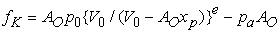(1)

where p0 and V0 are the initial pressure and volume of the gas in upper chamber respectively, and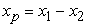(as shown in Figure 4).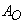is the effective pressurized area for outer diameters of the shock absorber. e is the polytropic index of gas inside the shock absorber. pa is the atmospheric pressure.

• Figure 4. Model of an aircraft landing gear

The resisting force fD due to the dynamic pressure is expressed as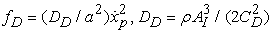(2)

where a is the orifice area ahole or axp (Figure 1 and Figure 2).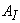is the effective pressurized area for inner diameters of shock absorber. CD is the discharge coefficient of orifice area, and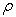is the density of fluid.

The total resisting force f of the shock absorber is expressed as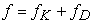(3)

When the lift acting on masses m1 and m2 is equal to the gravitational force acting on these masses, the equation of motion for the two-mass system shown in Figure 4, is expressed as

When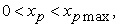and as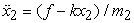(4)

When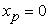or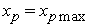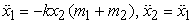(5)

Where m1 is the mass of aircraft including passengers, cargo, and fuel of one landing gear. m2 is the mass of tires and moving part of the shock absorber. k is the total spring constant of tires.

### 4. Optimization Problem

In the optimization, we deal with shock absorbers without metering pin (Figure 1) and with metering pin (Figure 2). The objective function of optimization is to minimize the maximum vertical acceleration of the aircraft mass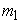. For the shock absorber without metering pin (Figure 1), the orifice areas are optimized for masses 130t and 85t. For shock absorber with metering pin (Figure 2), the tapered (Figure 3 (a), (b)), and parabolic (Figure 3 (c), (d)) profiles diameters are optimized at masses 130t and 85t. We minimize the maximum vertical acceleration of the aircraft while the metering pin passes through the hole (Figure 2) to obtain the diameters for the single-tapered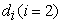and multi-tapered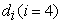profiles, and for the single-parabolic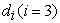and multi-parabolic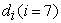profiles of the metering pin. We used MATLAB/Simulink 2015, and Global Optimization Toolbox to run simulations and optimizations in this work. ahole is the orifice area which does not depend on displacement xp, and axp is the orifice area which depends on displacement xp. Dxp is the metering pin diameter depending on displacement xp.

The parameters used in the calculations are, the effective pressurized area for inner and outer diameters of shock absorber piston tube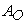= 0.123m2,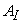=0.059 m2, the discharge coefficient of orifice area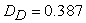kg･m3, the total spring constant of tires k = 4.3×106 kN/m, the mass of tires and moving part of shock absorber m2 = 1.8×103 kg, the polytropic index of gas inside shock absorber e = 1.1, the initial pressure of gas inside shock absorber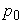= 106 Pa, the atmospheric pressure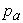= 1.01325×105 Pa, the aircraft landing speed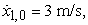the maximum displacement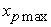= 0.65 m, and the area of the hole (Figure 1)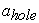= 0.0078 m2.

The Global Optimization toolbox from MATLAB 2015 are used in order to conduct the optimization. The GA solver creates optimum solutions for a single-objective minimization using the genetic algorithm.

The effective orifice area (Figure 2) is calculated using Eq. (6).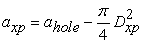(6)

Where, ahole is the hole area which the metering pin going through, and axp is the orifice area which subtract metering pin cross-section area depends on displacement xp from the hole area. Dxp is the metering pin diameter depending on displacement xp, is calculated in the section 4.2

The objective function to minimize the maximum vertical acceleration of aircraft mass, is expressed as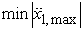(7)

The design variables are descripted and defined below in sections 4.1 and 4.2.

4.1. Shock Absorber without Metering Pin

This is a single design variable optimization problem. We optimize the orifice area ahole (Figure 1), which does not depend on displacement xp during landing. The objective function is to minimize the maximum vertical acceleration of the aircraft mass, when the mass is m1 = m1max=130t and m1 = m1min=85t.

4.2. Shock Absorbers with Metering Pin
4.2.1. Single-Tapered Profile

In this section, the orifice area axp, which depends on displacement xp during landing. The maximum vertical acceleration of the aircraft when the mass is m1 =130t (maximum) and 85t (minimum), is optimized to find the optimal solutions for diameters d1 and d2 (Figure 3(a)). The calculation for the diameters is expressed as Eq. (8).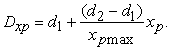(8)

4.2.2. Three-Taper Profile

The metering pin is divided equally into three-tapered profiles (Figure 3(b)). For the diameters di, the design variable numbers are i =1, 2, 3, 4. n is the equally divided number where n = 3 in this paper. The bottom of the first taper is the top of the next tapered profile, connecting to each other. The diameters of the three-tapered are expressed as Eq. (9), (10) and (11).

When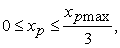the diameter is expressed as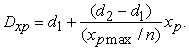(9)

When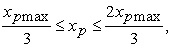the diameter is expressed as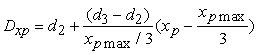(10)

When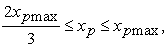the diameter is expressed as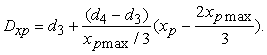(11)

4.2.3. Single-Parabolic Profile

In this section, we proposed a single-parabolic curved metering pin. There are three design variables in this optimization problem. The design variables d1, d2 and d3 are the top, middle and bottom diameters respectively (Figure 3(c)). The calculation for the diameter of the metering pin is expressed as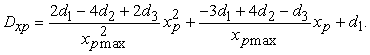(12)

4.2.4. Three-Parabolic Profile

Similar to section 4.2.2, the metering pin is divided into three parts, three-parabolic profiles equally (Figure 3(d)). The diameters di are the design variables, where i=1, 2, 3, 4, 5, 6, 7. The bottom of the first parabolic is the top of the next parabolic profile, connecting to each other. The diameters of the three parts parabolic are expressed as Eq. (13), (14) and (15).

Whenthe diameter is expressed as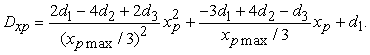(13)

Whenthe diameter is expressed as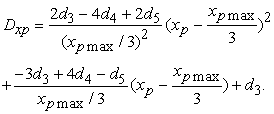(14)

Whenthe diameter is expressed as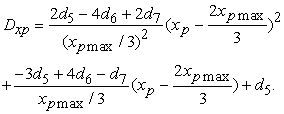(15)

### 5. Results and Comparisons

In in previous paper 12, the diameters of metering pin, displacements and the initial pressure of gas inside shock absorber are the design variables. In this study, we deal with the diameters of metering pin only.

We obtained the single-objective optimal results in Table 1 (optimal diameters) and Table 2 (optimal maximum vertical accelerations and orifice areas) for each type of metering pin in shock absorbers optimized at maximum and minimum masses m1=130t and 85t. In Table 2, there are also optimal orifice areas for shock absorber without metering pin at masses m1=130t and 85t. Using the results of the optimal diameters of metering pins in Table 1, we completed drawings of the optimal metering pins in Figure 5 and Figure 6.

In Figure 5, about the single-tapered metering pin, the shape of metering pins show taper geometry (Figure5 (b)) optimized at mass m1=85t, but for 130t (Figure 5 (a)), the shape becomes reverse taper. This means that the diameters not only becomes from smaller to greater, but also from greater to smaller too, along the displacement. For the three-tapered metering pins (Figure 5(c), (d)), the first step is taper, the second step is reverse taper, and the third is taper, for mass 130t (Figure 5 (c)). For 85t (Figure 5 (d)), the first step is reverse taper, the second step is taper, and the third is reverse taper. This means that the diameters (orifice areas) can be changed in detail compared to the single-tapered metering pin, for every case of the masses. From the optimization results, for the tapered metering pins, there are tapered and reverse tapered shapes depending on the masses, but for both the taper and reverse taper, the tapering angles are very small. This means that the diameters do not need to be changed widely along the displacement. For example, in Figure 5 (b), the metering pin shape is closer to a cylindrical column.

For the parabolic metering pin, if the middle diameter d2 is smaller or greater than top diameter d1 and bottom diameters d3 (Figure 3 (c)), the profiles will become concave or convex respectively. For example, Figure 6 (a) is convex, Figure 6 (b) is a half convex and a half concave. Figure 6 (c) and (d), are the optimum three-parabolic profiles, which have multi-convex and multi-concave geometry on the same metering pin for each case of masses. Although there are three parabolic profile parts, but there are actually seven design variables and six different convex or concave profiles that change the orifice areas finely and smoothly along the displacement.

Figure 7 and Figure 8 show the accelerations and resisting forces of the shock absorber without metering pins. Figure 7 (a) and Figure 8 (a) are the simulations of acceleration and resisting forces for 130t, 115t, 100t and 85t, using the results of optimization at 130t. Similarly, Figure 7 (b) and Figure 8 (b) are the simulations using the results of optimization at 85t.

Figure 9 compares the simulation for masses 130t, 115t, 100t and 85t by optimization results of the single-tapered and three-tapered metering pin. Figure 9(a) is optimization at 130t, Figure 9(b) is optimization at 85t. Comparing each mass, it is obvious that the maximum accelerations in the case of the three-taper metering pin (thin lines in Figure 9 (a) and (b)), are greater than the the case of single-taper metering pin (thick lines in Figure 9 (a) and (b)).

For the results of optimization at 85t (Figure 9 (b)), the interesting thing is that all the curves of the single-tapered and three-tapered metering pins for each case of different masses are almost fitting together, and the lines of the single-tapered and three-tapered metering pin are very smooth, because the optimum diameters solutions of the metering pin at 85t are very close (Figure 5 (c) and (d)), both of them have very small tapers, approximately the same as a cylindrical column.

Figure 10 compares the results of the single-parabolic and three-parabolic metering pin optimization at masses 130t and 85t. The acceleration curves of simulation of the single-parabolic using the results of optimization at masses 130t, have two big peaks (Figure 10 (a) thick lines), but the acceleration curves of the three-parabolic have more vibrations peaks (Figure 10 (a) thin lines). Figure 10 (b) is the comparison of the single-parabolic and the three-parabolic using the results of optimization at 85t. For the simulation curve of 130t using the results of optimization at 85t (Figure 10(b) thick and thin lines), although the acceleration increases toward the end, the simulation curve of three-parabolic metering pin at 85t (thin lines in Figure 10 (b)) is smoother and flatter than the curve of single-parabolic at 85t (thick lines in Figure 10 (b)).

• Figure 5. Diagrams of metering pins with single and three-tapered profile using optimal solutions
• Figure 6. Diagrams of metering pins with single and three parabolic profiles using optimal solutions
• Figure 7. Simulation of accelerations for shock absorber without metering pin
• Figure 8. Simulation of resistance forces for shock absorber without metering pin
• Figure 9. Comparison of single-taper and three-taper for optimization at 130t and 85t
• Figure 10. Comparison of single-parabolic and three-parabolic for optimization at 130t and 85t
• Figure 11. Comparison of three-parabolic and without metering pin for optimization at 130t and 85t

Figure 11 (a) is the comparison of the shock absorber without metering pin and the three-parabolic metering pin. The acceleration of the three-parabolic of 85t (Figure 11 (a) thick blue line) is 24.96% lower than the shock absorber without metering pin optimized at 130t (Figure 11 (a) thin blue line).

Figure 11 (b) is the comparison of the three-tapered and the three-parabolic metering pin. The simulation lines are very smooth, and they have favorable performances for all masses.

### 6. Efficiency Calculation for Shock Absorbers

The efficiency of the shock absorber is another important parameter to evaluate the performance. It can be determined from the load deflection curves. The efficiency is defined as the ratio of area under the load deflection curve to the area of the maximum load deflection. This is expressed mathematically by Eq. (16) below. This can be easily calculated with the numerical means of computing based plots, to accurately determine the efficiency of the shock absorber. Figure 12 is an example of the efficiency calculation. The efficiency of the shock absorber without metering pin at m1=130t is 86.96% (the red line), and the efficiency of the three-parabolic metering pin type shock absorber at m1=85t is 96.03% (the blue line).

• Figure 12. Efficiency for shock absorbers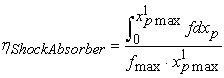(16)

The results of efficiency for each shock absorber are shown in Table 3. The efficiency of the three-parabolic metering pin optimized at 85t has the highest efficiency 96.03% (Table 3), but the shock absorber without metering pin optimized at 85t shows the lowest at 130t (57.38%, Table 3). In the case of the optimizations at 130t, all the efficiencies are greater than 75%, reaching almost up to 80%. In the case of the optimizations at 85t, all the efficiencies of 130t are lower, at around 60%.

In comparison, the shock absorber with three-parabolic shape metering pin shows the best performance, but the shock absorber optimized at 85t shows lowest efficiency in all the cases.

### 7. Conclusions

We focused on the single-objective optimization of passive shock absorbers to optimize the dimensions of the metering pin and the orifice area of hole. We obtained the optimal solutions for the proposed single-tapered, multi-tapered, single-parabolic and multi-parabolic metering pins at the maximum and minimum masses of aircraft. We also compared the performance and efficiency of the passive shock absorbers.

It is not possible to obtain ideal results to deal with all the aircraft masses as the different impact condition, but the metering pin can be shaped based on one particular important landing case to improve the other landing conditions. For the single-tapered metering pin, there are tapered or reverse tapered shapes depending on the mass it was optimized at. Nevertheless, whichever tapered or reverse tapered, their angles are very small. For single-parabolic metering pins, there are convex parabolic and concave parabolic shapes depending on the mass it was optimized at. Regardless, the parabolic curve is very small. This means that the diameters do not need to be changed drastically along the displacement of the shock absorber. For the three-taper and the three-parabolic, the diameters of the metering pins can be changed in detail along the displacement, while having better performance and higher efficiency during the landing.

### References

  Choi, Y. T., and Wereley, N. M., 2003, “Vibration Control of a Landing Gear System Featuring Electrorheological/Magnetorheological Fluids,” J. Aircraft, 40(3), pp. 432-439. In article View Article  McGehee, J. R., and Dreher R. G., Experimental Investigation of Active Loads Control for Aircraft Landing Gear, NASA-TP-2042, 1982. In article  Howell, W. E., McGehee, J. R., Daugherty, R. H., and Vogler, W. A., F-106B Airplane Active Control Landing Gear Drop Test Performance, SAE Technical Paper Series, 901911, 1990. In article View Article  Krüger, W., Design and Simulation of Semi-Active Landing Gears for Transport Aircraft, Mechanics of Structures and Machines, 30(4), 2002, pp. 493-526. In article View Article  Altuzarra, O., Hernandez, A., Salgado, O., and Angeles, J., Multiobjective Optimum Design of a Symmetric Parallel Schönflies-Motion Generator, ASME J. Mech, Des., 131(3), 2009, pp. (1002, 1-11). In article View Article  Wang, X., and Carl, U., Fuzzy Control of Aircraft Semi-active Landing Gear System, AIAA-99-0265, 1999. In article View Article  Kobayashi, M., Shi, F., and Maemori, K., 2009, Modeling and Parameter Identification of a Shock Absorber Using Magnetorheological Fluid, J. System Design and Dynamics, 3(5), pp. 804-813. In article View Article  Kobayashi, M., Shi, F., and Maemori, K., Parameter Identification and Optimization of Shock Absorber Using Magnetorheological Fluid, Journal of System Design and Dynamics, 7(3) , 265-277, 2013. In article View Article  Maemori, K., Tanigawa, N., Koganei, R., and Morihara, T., 2003, Optimization of a Semi-Active Shock Absorber for Aircraft Landing Gear, Proc. DETC’03 ASME 2003 Design Engineering Technical Conferences and Computer and Information in Engineering Conference, Chicago, IL, DETC2003/DAC-48765. In article View Article  Maemori, K., Tanigawa, N., and Shi, F., Optimization of a Semi-Active Shock Absorber Using a Genetic Algorithm, Proc. DETC’04 ASME 2004 Design Engineering Technical Conferences and Computer and Information in Engineering Conference, Salt Lake City, Utah, DETC2004-57115, 2004. In article  Shi, F., Tanigawa, N., Koganei, R., and Maemori, K., Optimum Trade-Off Charts Considering Mass Variation for the Design of Semi-Active and Passive Shock Absorbers for Landing Gear, Journal of Advanced Mechanical Design, Systems, and Manufacturing, 10(1), 1-15, 2016. In article View Article  Shi, F., Multi-objective Optimization of Passive Shock Absorber for Landing Gear. American Journal of Mechanical Engineering. 2019, 7(2), 79-86. In article View Article  JAL Practical Dictionary: Flight plan, Aircraft specifications and Ability Table. http://www.jal.com/ja/jiten/dict/p435.html In article

Published with license by Science and Education Publishing, Copyright © 2019 Fenghui Shi, Warren Isaac Anak Dean and Taikei SuyamaThis work is licensed under a Creative Commons Attribution 4.0 International License. To view a copy of this license, visit http://creativecommons.org/licenses/by/4.0/